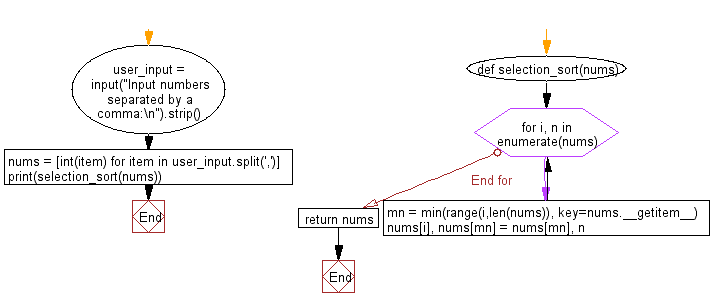﻿ Python: Sort a list of elements using Selection sort - w3resource# Python: Sort a list of elements using Selection sort

## Python Search and Sorting : Exercise-20 with Solution

Write a Python program to sort a list of elements using Selection sort.
According to Wikipedia "In computer science, selection sort is a sorting algorithm, specifically an in-place comparison sort. It has O(n2) time complexity, making it inefficient on large lists, and generally performs worse than the similar insertion sort".

Sample Solution:

Python Code:

``````def selection_sort(nums):
for i, n in enumerate(nums):
mn = min(range(i,len(nums)), key=nums.__getitem__)
nums[i], nums[mn] = nums[mn], n
return nums
user_input = input("Input numbers separated by a comma:\n").strip()
nums = [int(item) for item in user_input.split(',')]
print(selection_sort(nums))
```
```

Sample Output:

```Input numbers separated by a comma:
15, 79, 25, 37, 68
[15, 25, 37, 68, 79]
```

Flowchart:Python Code Editor:

What is the difficulty level of this exercise?

Test your Programming skills with w3resource's quiz.

﻿

## Python: Tips of the Day

The Zip() Function:

```>>> students = ('John', 'Mary', 'Mike')
>>> ages = (15, 17, 16)
>>> scores = (90, 88, 82, 17, 14)
>>> for student, age, score in zip(students, ages, scores):
...     print(f'{student}, age: {age}, score: {score}')
...
John, age: 15, score: 90
Mary, age: 17, score: 88
Mike, age: 16, score: 82
>>> zipped = zip(students, ages, scores)
>>> a, b, c = zip(*zipped)
>>> print(b)
(15, 17, 16)
```